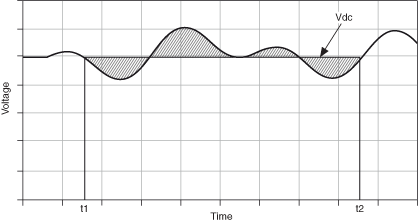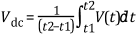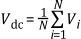# DC Level of a Signal

## LabVIEW 2018 Help

Edition Date: March 2018
Part Number: 371361R-01
View Product Info

### DOWNLOAD (Windows Only)LabVIEW 2016 HelpLabVIEW 2017 HelpLabVIEW 2018 HelpLabVIEW 2019 HelpLabVIEW 2020 Help

You can use DC measurements to define the value of a static or slowly varying signal. DC measurements can be both positive and negative. The DC value usually is constant within a specific time window. You can track and plot slowly moving values, such as temperature, as a function of time using a DC meter. In that case, the observation time that results in the measured value has to be short compared to the speed of change for the signal. The following figure shows an example DC level of a signal.The DC level of a continuous signal V(t) from time t1 to time t2 is given by the following equation.where t2 - t1 is the integration time or measurement time.

For digitized signals, the discrete-time version of the previous equation is given by the following equation.For a sampled system, the DC value is defined as the mean value of the samples acquired in the specified measurement time window.

Between pure DC signals and fast-moving dynamic signals is a gray zone where signals become more complex, and measuring the DC level of these signals becomes challenging.

Real-world signals often contain a significant amount of dynamic influence. Often, you do not want the dynamic part of the signal. The DC measurement identifies the static DC signal hidden in the dynamic signal, for example, the voltage generated by a thermocouple in an industrial environment, where external noise or hum from the main power can disturb the DC signal significantly.

Not Helpful